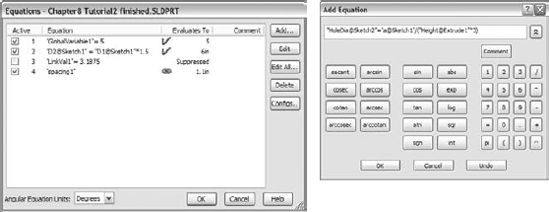# Chapter 9. Using Equations

IN THIS CHAPTER

• Understanding equations

• Global variables

• Expressions

• Tutorial: Equations

Parametric sketch relations are not the only way to drive dimensions with intelligence. You can also use equations, link values, and global variables. Equations help you to create simple or complex mathematical relations between dimensions. Link values are essentially a quick way of making two dimensions equal. Global variables can be used in equations like other dimension names. These three techniques are all very similar and related to one another in the interface, but are used in different ways in different situations.

Equations are a very powerful extension of the set of parametric power tools that SolidWorks offers to users. If you are not accustomed to driving dimensions with equations, then you may find it challenging to get the order of equations correct so that dimensions are not changed multiple times when more than a few equations are involved.

# Understanding EquationsFigure 9.1. The Equations interface

Using the Equations interface, you can turn off equations temporarily by deselecting the Active check box in front of the equation. Equations can also be deactivated by a design table. I will discuss design tables in more detail in Chapter 10, which discusses ...

Get SolidWorks® 2007 Bible now with the O’Reilly learning platform.

O’Reilly members experience live online training, plus books, videos, and digital content from nearly 200 publishers.